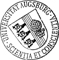## Local multigrid on adaptively refined meshes and multilevel preconditioning with applications to problems in electromagnetism and acoustics

• We consider local multigrid methods for adaptive finite element and adaptive edge element discretized boundary value problems as well as multilevel preconditioned iterative solvers for the finite element discretization of a special class of saddle point problems. The local multigrid methods feature local smoothing processes on adaptively refined meshes and are applied to adaptive P1 conforming finite element discretizations of linear second order elliptic boundary value problems and to adaptive curl-conforming edge element approximations of H(curl)-elliptic problems and the time-harmonic Maxwell equations. On the other hand, the multilevel preconditioned iterative schemes feature block-diagonal or upper block-triangular preconditioned GMRES or BiCGStab applied to the resulting algebraic saddle point problems and preconditioned CG applied to the associated Schur complement system. As technologically relevant applications of the above methods, we consider the numerical simulation ofWe consider local multigrid methods for adaptive finite element and adaptive edge element discretized boundary value problems as well as multilevel preconditioned iterative solvers for the finite element discretization of a special class of saddle point problems. The local multigrid methods feature local smoothing processes on adaptively refined meshes and are applied to adaptive P1 conforming finite element discretizations of linear second order elliptic boundary value problems and to adaptive curl-conforming edge element approximations of H(curl)-elliptic problems and the time-harmonic Maxwell equations. On the other hand, the multilevel preconditioned iterative schemes feature block-diagonal or upper block-triangular preconditioned GMRES or BiCGStab applied to the resulting algebraic saddle point problems and preconditioned CG applied to the associated Schur complement system. As technologically relevant applications of the above methods, we consider the numerical simulation of Logging-While-Drilling tools in oil exploration and the numerical simulation of piezoelectrically actuated surface acoustic waves.• Dokument_1.pdfAuthor: Ronald H. W. HoppeGND, Xuejun Xu, Huangxin Chen urn:nbn:de:bvb:384-opus4-11489 https://opus.bibliothek.uni-augsburg.de/opus4/1388 Preprints des Instituts für Mathematik der Universität Augsburg (2010-05) Preprint English Universität Augsburg University of Houston, Chinese Academy of Sciences 2010/04/26 local multigrid methods; adaptively refined meshes; multilevel preconditioners; Logging-While-Drilling; surface acoustic waves Mehrgitterverfahren; Akustische Oberflächenwelle; Elliptisches Randwertproblem Mathematisch-Naturwissenschaftlich-Technische Fakultät Mathematisch-Naturwissenschaftlich-Technische Fakultät / Institut für Mathematik Mathematisch-Naturwissenschaftlich-Technische Fakultät / Institut für Mathematik / Lehrstuhl für Numerische Mathematik 5 Naturwissenschaften und Mathematik / 51 Mathematik / 510 MathematikDeutsches Urheberrecht mit Print on Demand